## Cipher## Jan 31, 2017

### CNN 318 153 bible reference with Lucy and Saccorhytus "our earliest ancestor"

3.14 and 0.318 are special numbers related to the circumference of a circle
22/7 = 3.142857... is the closest approximation of Pi with small numbers (333/106 = 3.141509... or 355/113 = 3.1415929...)
7/22 = 0.318 which is sort of inverse Pi
You can calculate the circumference of any circle just by dividing 2x radius or diameter by 0.318

So if we decided for our circle to have a radius of 8 it would be (8x2)/0.318 = 50.3144654...
This picture is from online calculator that calculates the circumference like we are taught in school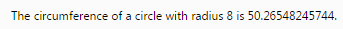318 is also one of important biblical numbersGenesis 14:14 is 351st verse in the Old Testament

The second coming of Jesus Christ is mentioned 318 in the New Testament
And the most important part is the miraculous catch of 153 fish in John 21:11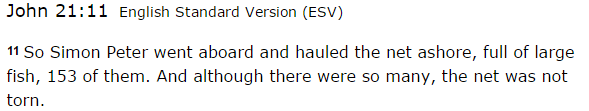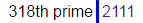21*11 = 231 (octal of 153)

Also 1122nd minute of the day leaves 318 minutes

These are the 2 CNN stories that are connected with 318 and 153
http://edition.cnn.com/2016/08/29/health/lucy-early-human-ancestor-cause-of-death/index.html
http://edition.cnn.com/2017/01/30/health/earliest-human-ancestor-deuterostome-saccorhytus-history-study/index.html

Yesterday on January 30th CNN released a story saying that microfossils from China have revealed our "earliest ancestor" which supposedly lived 540 million years ago and as you scroll through the story they have a related story on the left, which gave me the first clue, then I saw how they mention that Lucy our 'early ancestor' died 3.18 million years ago

This is how they showed related articleThis is from August 30th 2016 saying that Lucy provided us with new insight about our 'early ancestors' 3.18 million years agoFrom Lucy's article to January 30th is 153 days of course also it is 21 weeks and 6 days a lot like 216 (6x6x6)
Saturn = 19+1+20+21+18+14 = 93 (Ordinal)
Thirtieth of January = 2+8+9+9+2+9+5+2+8 + 6+6 + 1+1+5+3+1+9+7 = 93 (Reduced)three hundred eighteen = 2+8+9+5+5 + 8+3+5+4+9+5+4 + 5+9+7+8+2+5+5+5 = 113 (Reduced)
one hundred fifty three = 6+5+5 + 8+3+5+4+9+5+4 + 6+9+6+2+7 + 2+8+9+5+5 = 113 (Reduced)
one five three = 50+40+5 + 6+9+700+5 + 100+8+80+5+5 = 1013 (Jewish)
miraculous catch of fish = 4+9+9+1+3+3+3+6+3+10 + 3+1+2+3+8 + 6+6 + 6+9+10+8 = 113 (S Exception)
fish = 6+9+90+8 = 113 (Jewish)
Second coming of Jesus Christ = 1+5+3+6+5+4 + 3+6+4+9+5+7 + 6+6 + 1+5+1+3+1 + 3+8+9+9+1+2 = 113 (Reduced)
November 3rd is written 11/3 in some parts of the world
November Third = 14+15+22+5+13+2+5+18 + 20+8+9+18+4 = 153 (Ordinal)
Note that this story was released on 30th day of the yearSaturn = 10+1+2+3+9+5 = 30 (S Exception)
Saturn's orbital period is between 28-30 years (360 months - Three hundred sixty = 227)
Jesus Christ = 10+5+19+21+19 + 3+8+18+9+19+20 = 151 (Ordinal)It's all very interesting when you consider how 151 is 227 in octal system, 318th prime is the verse in which Jesus Christ catches 153 fish and we have "Anti-Pi" and Pi meeting there

Story about Lucy was released on a date with 74 numerology which even more corresponds to Jesus or Lucifer (74)
8/30/2016 - 8+30+20+16 = 74 (Jesus = 74) also Lucy was 'found' in 1974
Jesus is the son of 'God' = 7+6+4 =  17 - Reduced  (son of the God 'EL' = 5+12 = 17)
If you turn 7 you can get L and the letters E and L are in the same positions in English and in Hebrew alphabet
7th prime number is 17 which is the reason it has great significance and the 17th triangular number is 153
17th tetrahedral number is 969 (science says that speed of Saturn is 9.69km/s)
It's interesting that 107 converted into Octal system becomes 153
Also another number related to Saturn is 119 which is 7x17 also 156th prime number is 911
Saturn = 45+1+20+21+18+14 = 119 (Francis Bacon)
God Saturn = 7+15+4 + 19+1+20+21+18+14 = 119 (Ordinal)

Back to the story,  the cycle isn't complete with this story coming out today, from what I'm seeing they are pointing to the release of the new Alien movie on May 19th 2017
This is how they opened the post from today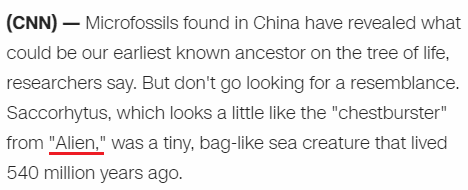There's many reasons this is interesting
May Nineteenth = 13+1+25 + 14+9+14+5+20+5+5+14+20+8 = 153 (Ordinal)
5/19/17 - 5+19+17 = 41
1/30/2017 - 1+30+2+0+1+7 = 41
Alien = 1+12+9+5+14 = 41 (Ordinal)
13th prime number is 41 and 13 is also the number related to Saturn
13th Fibonacci number is 233 which is also 351 in Octal numbering system
God = 7+15+4 = 26 - Ordinal (26th triangular number is 351)
(Something interesting I found today - 13th perfect number is 314 digits long)

The movie is called "Alien: Covenant" https://en.wikipedia.org/wiki/Alien:_Covenant
Alien: Covenant = 1+3+9+5+5 + 3+6+4+5+5+1+5+2 = 54 (Reduced)
They are saying that this creature lived 540 million years ago?
Alien: Covenant = 1+12+9+5+14 + 3+15+22+5+14+1+14+20 = 135 (Ordinal)
135+531 = 666They are giving a tribute on a date with 93 gematria to a date that leaves 226 days in the year and both are numerological match

Note that 318th day in the year is November
November Fourteenth = 14+15+22+5+13+2+5+18 + 6+15+21+18+20+5+5+14+20+8 = 226 (Ordinal)
Two hundred twenty-six = 2+5+6 + 8+3+5+4+9+5+4 + 2+5+5+5+2+7+1+9+6 = 93 (Reduced)
November Fourteenth = 40+50+700+5+30+2+5+80 + 6+50+200+80+100+5+5+40+100+8 = 1506 (Jewish)
One hundred fifty six = 15+14+5 + 8+21+14+4+18+5+4 + 6+9+6+20+25 + 19+9+24 = 226 (Ordinal)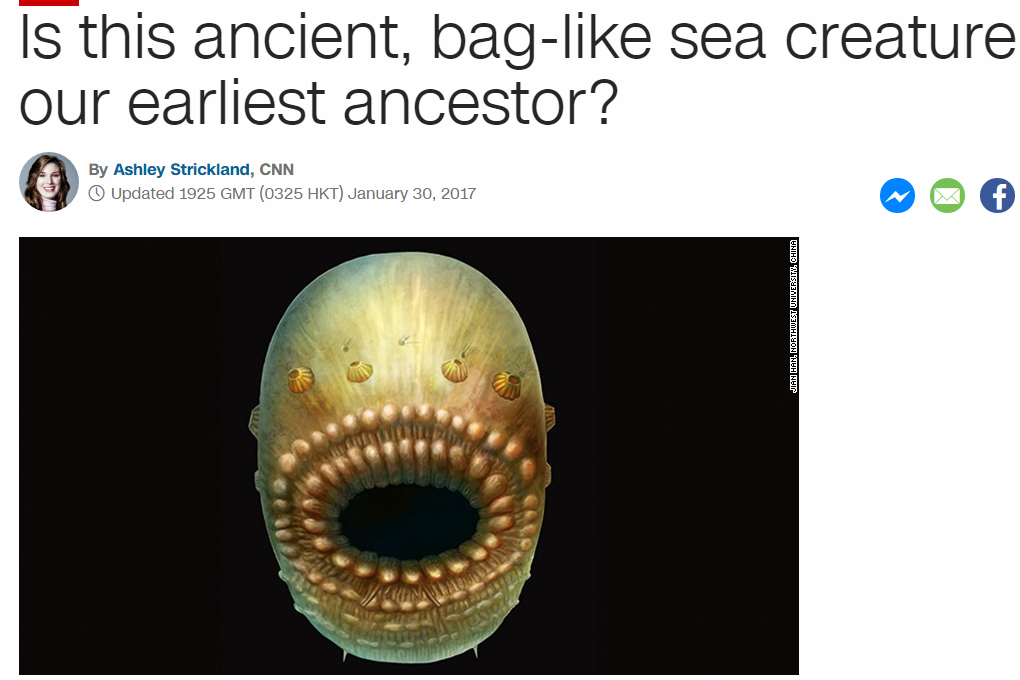They compare it to "Chestburster" from Alien
chestburster = 3+8+5+100+200+2+300+90+100+200+5+90 = 1103 (English)
Saccorhytus = 1+1+3+3+6+9+8+7+2+3+1 = 44 (Reduced)
They end their post with this
Funny to think, but in half a billion years, a lot of evolutionary changes can take place = 330 (S Exception)

Mithras = 30+9+100+8+80+1+90 = 318 (Jewish) - Roman Sun God before they adopted Christianity

From 11/14 (318th day) to 1/30/2017 is 77 days, which is interesting and shows why there's at least 3 stories today with 318 coding
Christ = 77 (7x7 = 49)
49th prime is 227 - Miraculous catch of fish - Pi (227) and "inverse Pi" (318) coding
Twenty-two divided by seven = 314 (Ordinal)
Also 3/18 is 77th day of the yearSome unrelated finds
314 in Roman numerals - CCCXIV = 3+3+3+6+9+4 = 28 (Reduced)
28 is 2nd perfect number also 7th triangular number is 28The number of Skull and Bones is 322 (322+232+223 = 777)
The number for Saturn in Jewish gematria is 511 (511+151+115 = 777)

Adding all combinations of 314 we arrive at 1776 the creation of Bavarian Illuminati and America's declaration of Independence
314+341+143+134+431+413 = 1776